The word ‘LED’ is an abbreviation for ‘Light-Emitting Diode’. So a LED is a  diode that emits light. So it is actually a light-emitting diode. However, the LED does have other properties than the old-fashioned light bulb.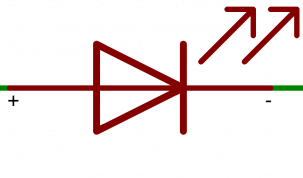The symbol of a LED

# Polarity

Like a diode, an LED must be connected in the correct orientation. If it is connected in the wrong direction, it will not conduct current, and therefore will not emit light. However, just like a diode, the led will not break.

Most LEDs will have the anode(+) recognized because this pin is longer than the cathode(-), also the led will have a flat side at the side of the cathode(-).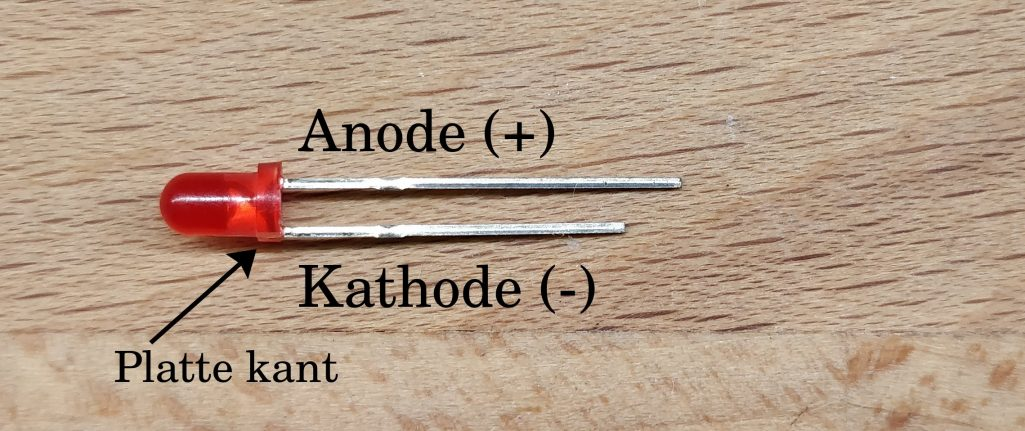To turn on the LED, the anode(+) must be connected to the positive side of the power source. The cathode(-) will have to be connected on the negative side.

# How many volts?

The voltage we have to put on a LED is a bit confusing. This is because, with LEDs, the current is especially important. However, at least the passage voltage (more information) must be exceeded. With a typical 3mm led, this will be around 2 volts. Theoretically, an LED has no maximum voltage.

So the voltage we put on the led does not need to be regulated, but we will have to regulate the current. For most 3mm LEDs, the maximum current will be around 20 milliamps. We can easily adjust the current using a resistor. For this, we use the circuit shown below. In this circuit, the VCC is the positive side of the voltage source, and GND is the negative side.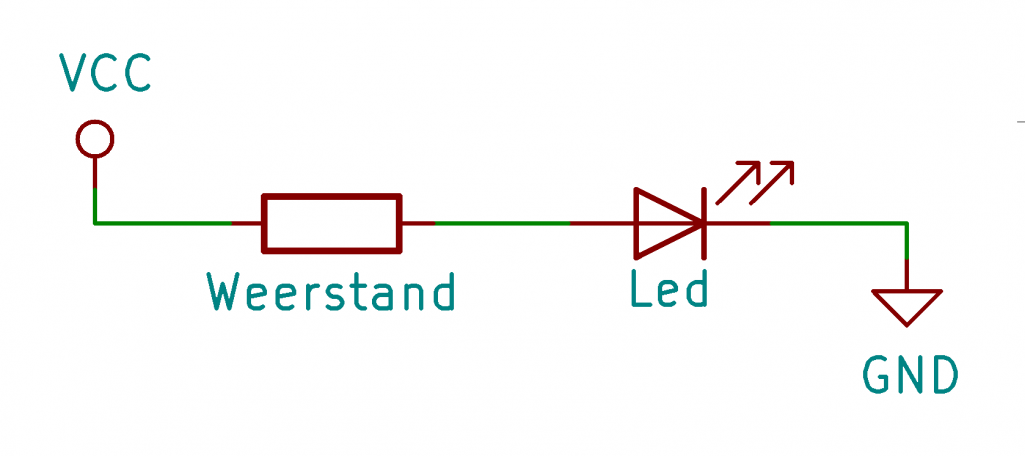To calculate which value we want to use for the resistor, we can apply Ohm’s law. According to this law the following applies: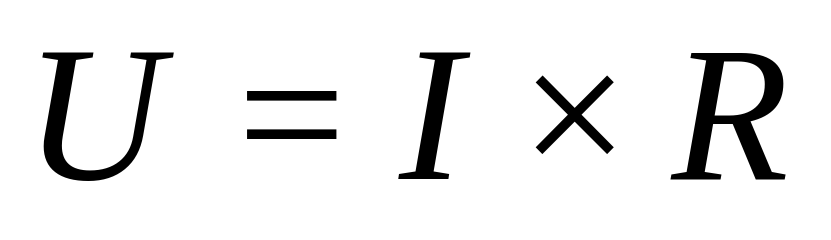When we divide the left and right side of the =-sign by I, we get the following formula: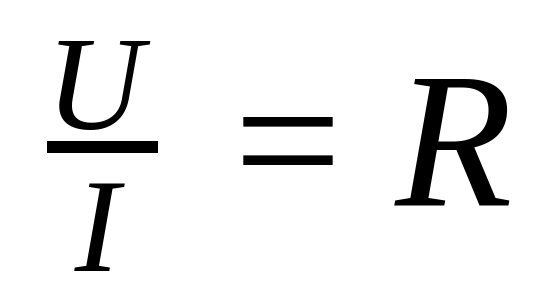In physics we shorten voltage with U, current with I and resistance with R. So the above formula actually means the following: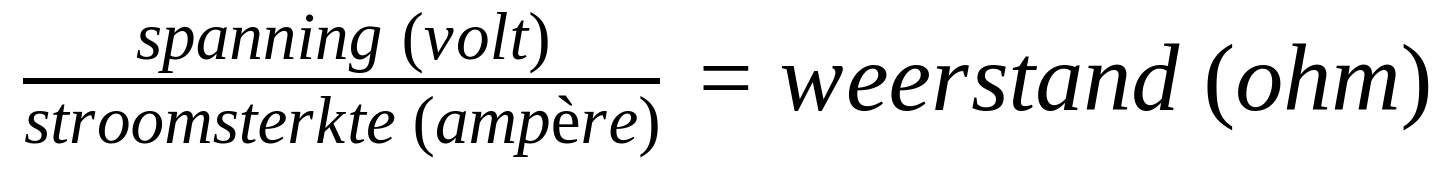In this formula, we can fill in the voltage of our voltage source in volts, and we can fill in the desired current in amperes (1 ampere = 1000 milliamps). The result of this formula will be the value we want to use for our resistor, calculated in ohms. However, we won’t be able to find the resistor with exactly this value, so we will have to choose the resistor with the value that is as close as possible.

## Internal resistence

In our workshop, we often put LEDs directly on a button cell battery. But… …L at some point, the LED will break anyway… Without a resistor, too much current runs through the LED. According to the theory given above, the led should of course break, but in reality, this does not happen. This is because every voltage source has an internal resistance. In fact, every component, even our cords, has a resistor. So this means that even when we don’t use a resistor, our circuit will always have a resistor (in ohms). However, for many components, the resistance value is negligible, as it is very small.

However, the internal resistance of a button cell battery is quite high. When we directly connect a LED to it, there will be little current flowing, and as a result, the LED will not break. Also when we connect a LED directly to an Arduino, it will not break, this is because the Arduino also has a large resistor. However, for the lifetime of both the LED and the Arduino, it is recommended to measure if there is not too much current running through the LED.

A LED will, when we connect it directly to a LiPo battery, however, break down immediately. LiPo batteries have a very low internal resistance, meaning a lot of current will flow through them.

# Cheat Sheet

Of course, it is a lot of work to recalculate the resistance value we need each time. For this reason, we have already calculated the resistance values you will need for a typical 3mm led. To be on the safe side, we have calculated these values to provide the LED with 15 milliamps.

3,0 volt  –  200 ohm
3,3 volt  –  220 ohm
5,0 volt  –  330 ohm

# Its a circuit!

In this article, we learned how to work with a LED. But besides knowledge, experience is also very important. In the lab ‘How do you design a circuit?’ we learn how to design a circuit, in this project the knowledge we learned with this article also comes back.